Design of Experiments Guide > Prospective Sample Size and Power > Reliability Demonstration Calculator > Statistical Details for the Reliability Demonstration Calculator
Publication date: 07/30/2020

## Statistical Details for the Reliability Demonstration Calculator

The reliability demonstration depends on the assumed failure time distribution with scale parameter σ. The reliability standard, or probability of survival at time t and location μ is stated as follows: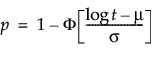where μ is solved for using the following: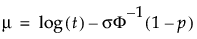.

To calculate sample size and the size of the test, the probability of survival at time t is posed as a hypothesis test: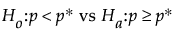where p* is the standard probability of survival at time t*.

We want to test the hypothesis at the α level or as follows:

α = Pr(k or few failures | H0 true).

Since the test is of n independent units, the number of failures has a binomial (n, p) distribution where p is the probability of a unit failing before time t. Therefore, we can express α as a function of t and n: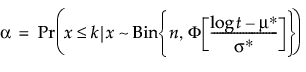where μ* and σ* are from the assumed reliability standard.

Properties of the binomial and beta distributions result in being able to solve for t using: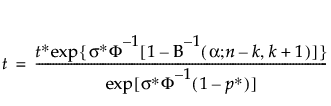For n, Brent’s method is used to find the root of: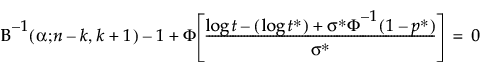where:

B1(α; n k, k + 1) is the α quantile of the Beta(n k; k + 1) distribution

and Φ() is the cumulative distribution function of the assumed failure time distribution.